If you have a related question, please click the "Ask a related question" button in the top right corner. The newly created question will be automatically linked to this question.

# OPA132: Simulating the PSRR of the OPA132 with Tina

Part Number: OPA132
Other Parts Discussed in Thread: OPA170, TINA-TI

Hi engineers,

I have a problem about using Tina to simulate an OPA's DC PSRR.

I learned the PSRR of OPA in the Precision Lab. Below is the result of the Tina simulation in the Precision Lab tutorial.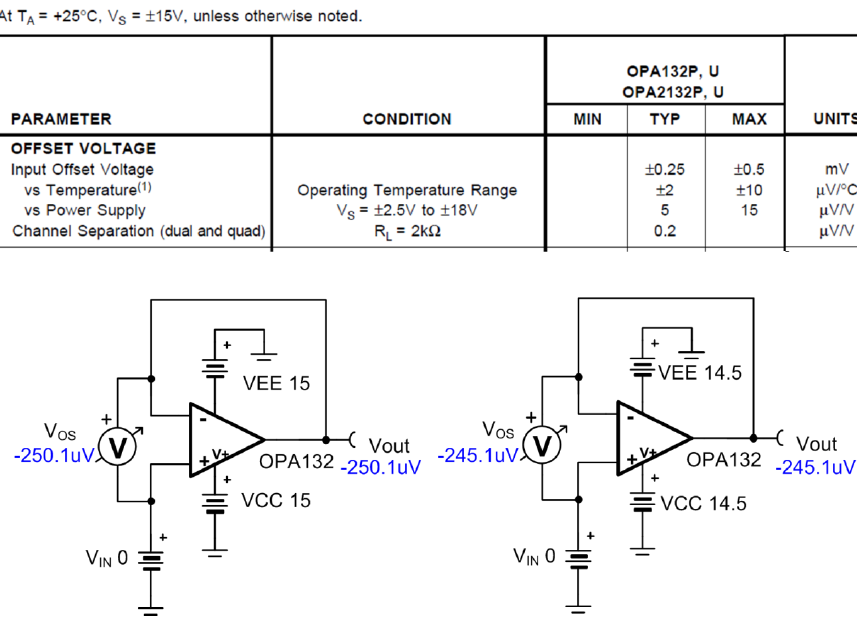I also chose OPA132 and simulated it with Tina. Here are the circuits and results I took.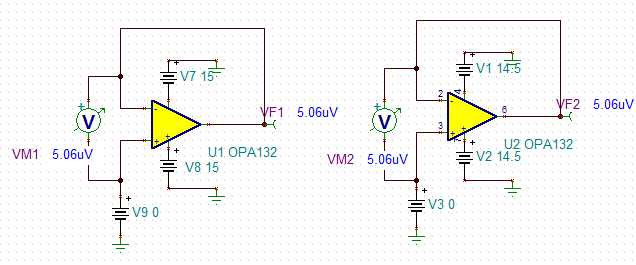As you can see, the power supply was changed (a total of 1V changes), and Vos did not change, which is different from the tutorial results in the Precision Lab.

In addition, use the same circuit, but the op amp is replaced by OPA111, Vos still has no change. As shown below.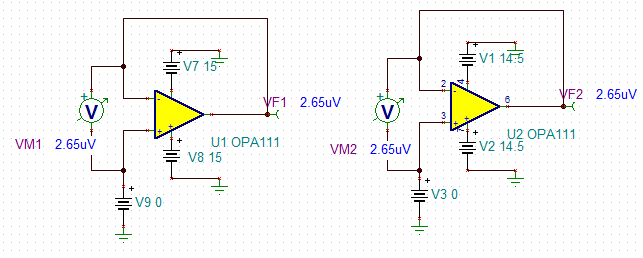However, still use this circuit, the op amp is replaced with OPA170, the power supply changes, the op amp Vos changes.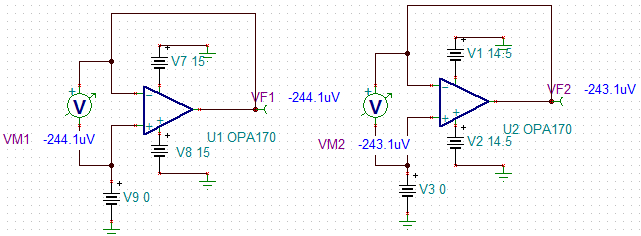Below is the DATASHEET of the op amp OPA170. It can be seen that the change in Vos is consistent with that described in DATASHEET.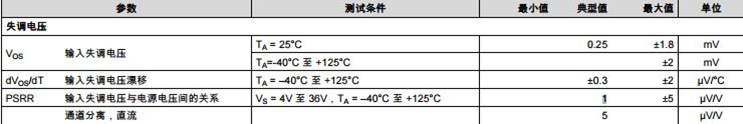Therefore, it can be verified that the circuit taken should be no problem.

Why does the Tina simulation of the OPA132 in the Precision Lab get the correct results, and why does the Tina simulation I took not get the correct results?